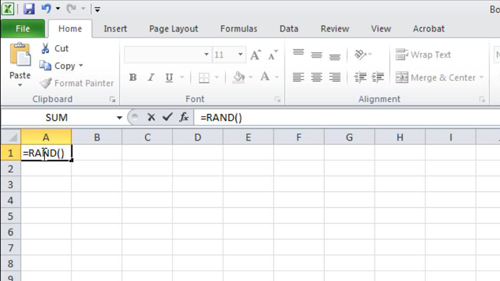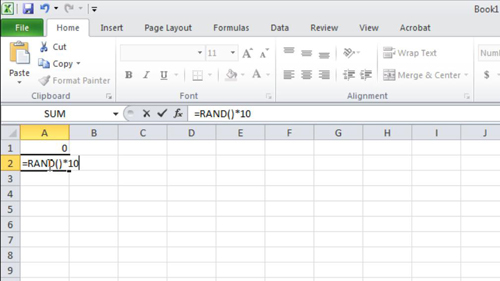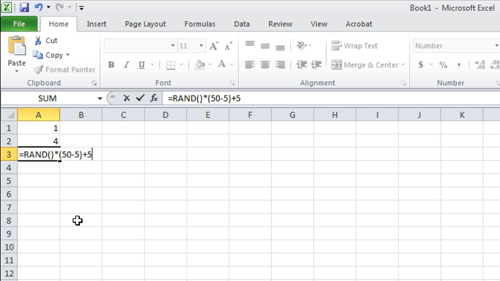## How to Generate Random Numbers in Excel

In this tutorial, you are going to learn how to use the Excel random number generator.

Step # 1 – Generate a random number from 0 to 1

First, open a new spreadsheet. Click in cell A1 (or wherever you want to insert a random number). Type “=RAND( )” in the cell. This will generate a random number from 0 to 1.Step # 2 – Generate a random number from 0 to n

Click another cell (here the A2 cell). If you want to generate a number from 0 to any number you want, type “=RAND( )*”. Then a number you want to generate up to, here we are entering 10. This will generate any number from 0 to 10.Step # 2 – Generate a random number from a given range

Click into another cell (here A3). If you want to generate a number in a given range, type “=RAND( )*([top end number]-[low end number])+[low end number]”. The example we are using here is “=RAND( )*(50-5)+5”. This will generate any number from 5 to 50. That’s it, you now know how to use the Excel random number generator.